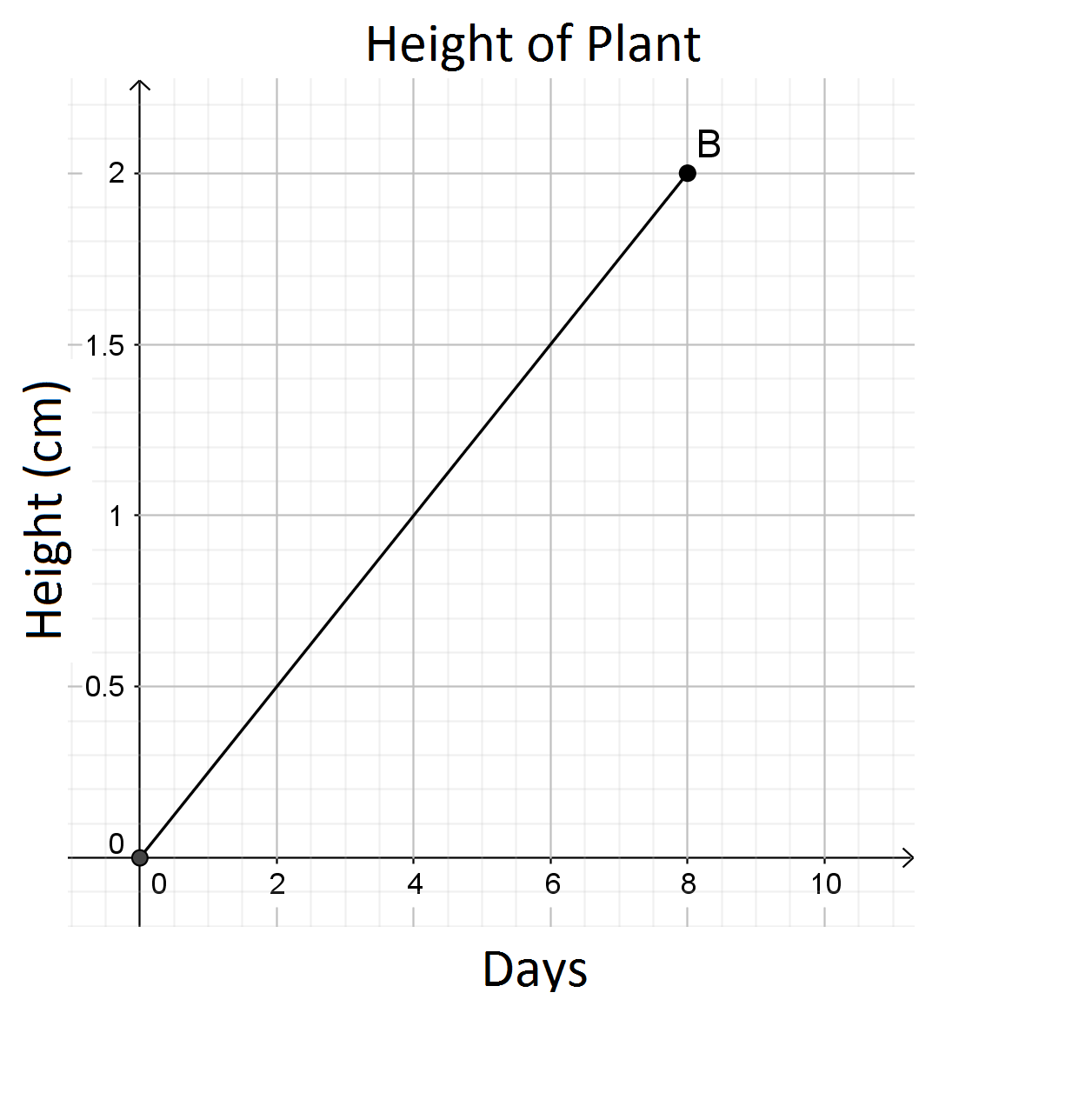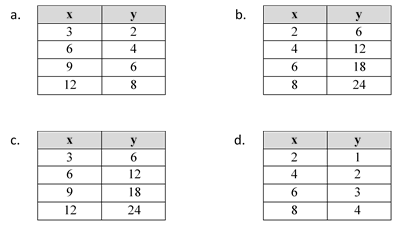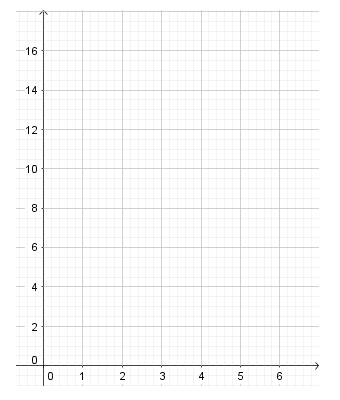# Proportional Relationships

## Objective

Make connections between the four representations of proportional relationships (Part 1).

## Common Core Standards

### Core Standards

?

• 7.RP.A.2 — Recognize and represent proportional relationships between quantities.

• 7.RP.A.2.A — Decide whether two quantities are in a proportional relationship, e.g., by testing for equivalent ratios in a table or graphing on a coordinate plane and observing whether the graph is a straight line through the origin.

• 7.RP.A.2.B — Identify the constant of proportionality (unit rate) in tables, graphs, equations, diagrams, and verbal descriptions of proportional relationships.

• 7.RP.A.2.C — Represent proportional relationships by equations. For example, if total cost t is proportional to the number n of items purchased at a constant price p, the relationship between the total cost and the number of items can be expressed as t = pn.

• 7.RP.A.2.D — Explain what a point (x, y) on the graph of a proportional relationship means in terms of the situation, with special attention to the points (0, 0) and (1, r) where r is the unit rate.

?

• 6.RP.A.3

## Criteria for Success

?

1. Identify the constant of proportionality in graphs, equations, and tables.
2. Represent and analyze proportional relationships in graphs, equations, and tables.

## Tips for Teachers

?

Lessons 10 and 11 pull together all the representations of proportional relationships – written description, table, graph, and equation – that students have been studying since the beginning of the unit. As needed, review any one representation that students may be stuck on, but continue to engage them in looking at multiple representations at a time in order to strengthen the connections between them.

#### Remote Learning Guidance

If you need to adapt or shorten this lesson for remote learning, we suggest prioritizing Anchor Problems 1 and 3 (can be done independently). Find more guidance on adapting our math curriculum for remote learning here.

#### Fishtank Plus

• Problem Set
• Student Handout Editor
• Vocabulary Package

## Anchor Problems

?

### Problem 1

A scientist plants a seed in a laboratory and tracks the growth of the plant. The height of the plant in centimeters is proportional to the number of days since it was planted.

1. Explain what point B represents in context of the situation.2. At what rate is the plant growing? Indicate this as a point on the graph.
3. Write an equation that represents the relationship between days and height.
4. If the plant continues to grow at this rate, how tall will it be after 12 days?
5. If the plant continues to grow at this rate, when will it be 6 cm tall?

### Problem 2

Match each table to its equation.1.   ${y = {1 \over 2}x}$
2.   ${y = {2 \over 3}x}$
3.   ${y = 2x}$
4.   ${y=3x}$

### Problem 3

The cost you pay for limes is proportional to the number of limes you buy. Four different stores sell limes for different amounts, as shown in the graphs, table, and equation below. Which store should you go to if you want to pay the least amount for limes? Explain your answer.## Problem Set

?The following resources include problems and activities aligned to the objective of the lesson that can be used to create your own problem set.

?

The width of a small book measures 6 inches, or approximately 15 centimeters. You know that the relationship between inches and centimeters is proportional, but you can’t remember the conversion rate between them.

Let $y$ represent the number of centimeters and $x$ represent the number of inches. Represent the relationship in the coordinate plane below, and then write an equation that approximates the relationship between inches and centimeters.?